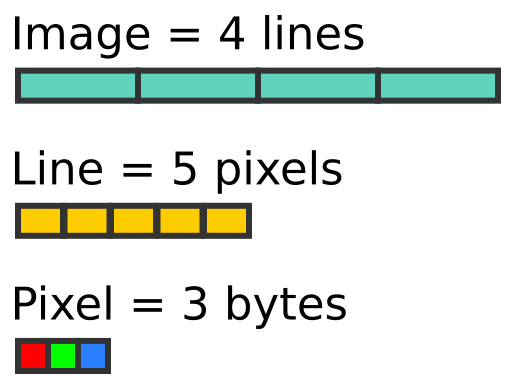# Python informer

Improve your Python coding skills

## Image processing with numpy

In this section we will learn how to use numpy to store and manipulate image data. We will use the Python Imaging library (PIL) to read and write data to standard file formats.

If you want to learn more about numpy in general, try the other tutorials.

Before trying these examples you will need to install the numpy and pillow packages (pillow is a fork of the PIL library).

## Creating RGB Images

Here is a 5 by 4 pixel RGB image:The image contains 4 lines of pixels. Each line of pixels contains 5 pixels. Each pixel contains 3 bytes (representing the red, green and blue values of the pixel colour):RGB images are usually stored as 3 dimensional arrays of 8-bit unsigned integers. The shape of the array is:

height x width x 3.


Here is how we create an array to represent a 5 pixel wide by 4 pixel high image:

{% highlight python %} import numpy as np

width = 5 height = 4

array = np.zeros([height, width, 3], dtype=np.uint8) {% endhighlight %}

Notice that the first dimension is the height, and the second dimension is the width. That is because the data is ordered by lines, then each line is ordered by pixels, and finally each pixel contains 3 byte values for RGB. Each colour is represented by an unsigned byte (numpy type uint8).

Now let’s fill the array with orange pixels (red=255, green=128, blue=0). We use slices to do this, the three values are broadcast across all the rows and columns of the array:

{% highlight python %} array[:,:] = [255, 128, 0] {% endhighlight %}

## Saving an RGB image using PIL

Now we can use fromarray to create a PIL image from the numpy array, and save it as a PNG file:

{% highlight python %} from PIL import Image

img = Image.fromarray(array) img.save(‘testrgb.png’) {% endhighlight %}

In the code below we will:

• Create a 200 by 100 pixel array
• Use slice notation to fill left half of the array with orange
• Use slice notation to fill right half of the array with blue

Here is the complete code:

{% highlight python %} import numpy as np from PIL import Image

array = np.zeros([100, 200, 3], dtype=np.uint8) array[:,:100] = [255, 128, 0] #Orange left side array[:,100:] = [0, 0, 255] #Blue right side

img = Image.fromarray(array) img.save(‘testrgb.png’) {% endhighlight %}

And here is the image:## Creating RGBA images

An RGBA image has 4 channels (unlike an RGB image that has only 3). The fourth channel is an alpha channel. An alpha value of 255 will make the pixel fully opaque, value 0 will make it fully transparent, values in between will make the pixel partly transparent.

In the code below we create an RGBA image, initially setting the same blue and orange areas as before, with and alpha value of 255. We then loop over the image changing the alpha value of each pixel to be equal to its x coordinate. This means that the pixels on the left side of the image will be transparent, and the pixels at the right will be almost fully opaque. The transparency varies smoothly from left to right.:

{% highlight python %} import numpy as np from PIL import Image

array = np.zeros([100, 200, 4], dtype=np.uint8) array[:,:100] = [255, 128, 0, 255] #Orange left side array[:,100:] = [0, 0, 255, 255] #Blue right side

# Set transparency depending on x position

for x in range(200): for y in range(100): array[y, x, 3] = x

img = Image.fromarray(array) img.save(‘testrgba.png’) {% endhighlight %}

And here is the image:## Creating greyscale images

Greyscale images are handled slightly differently. Because there is only one channel, there is no need to create a 3 dimensional array, you should use a 2 dimensional array instead:

{% highlight python %} import numpy as np from PIL import Image

array = np.zeros([100, 200], dtype=np.uint8)

# Set grey value to black or white depending on x position

for x in range(200): for y in range(100): if (x % 16) // 8 == (y % 16) // 8: array[y, x] = 0 else: array[y, x] = 255

img = Image.fromarray(array) img.save(‘testgrey.png’) {% endhighlight %}

In this case, we have created a chequerboard image:You can read an image using the PIL open function, and convert it to an array using the numpy array function. Here, we read the images that were created previously, and print their numpy shape:

{% highlight python %} import numpy as np from PIL import Image

img = Image.open(‘testrgba.png’) array = np.array(img) print(array.shape) # (100, 200, 4)

img = Image.open(‘testrgb.png’) array = np.array(img) print(array.shape) # (100, 200, 3)

img = Image.open(‘testgrey.png’) array = np.array(img) print(array.shape) # (100, 200) {% endhighlight %}

You can manipulate the image data and write it back out to file. For example, this code inverts a greyscale image (swapping black and white). and saves it back:

{% highlight python %} import numpy as np from PIL import Image

img = Image.open(‘testgrey.png’) array = np.array(img)

array = 255 - array

invimg = Image.fromarray(array) invimg.save(‘testgrey-inverted.png’) {% endhighlight %}